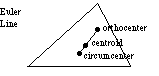index: click on a letter A B C D E F G H I J K L M N O P Q R S T U V W X Y Z A to Z index index: subject areas numbers & symbols sets, logic, proofs geometry algebra trigonometry advanced algebra & pre-calculus calculus advanced topics probability & statistics real world applications multimedia entrieswww.mathwords.com about mathwords website feedback

 Euler Line The line segment that passes through a triangle’s orthocenter, centroid, and circumcenter. These three points are collinear for any triangle. In addition, the distance from the orthocenter to the centroid is twice the distance from the circumcenter to the centroid. Note: Euler is pronounced "Oiler".See also